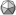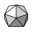Home/ www.icosaedro.it#Smartphone Battery Recharge Time

Updated: 2022-10-13

Or how to recharge the battery of your smartphone up to, let say, 90% by using a cheap constant voltage power adapter and so prolonging the life of your smartphone.

As many on the Internet suggest, fully recharging the Li-ion battery of your smartphone is not the best way to prolong its life. The suggested optimal top re-charge level varies between 85% and 90% depending on the author. Here I'll assume 90% as the top recharge level for my Xiaomi Redmi 10. Since the device comes with only a cheap constant voltage power adapter, the only way to stop the recharge to a specific level is by timing the recharge time. The problem is, how much time it will require to re-charge if I start from a given lower level?

By monitoring the time it takes to reach 90% of the full charge starting from different lower levels, I first collected all the data and I drawn a time-vs-level curve. From that heuristic curve, I then created the following model for the power adapter and battery set:Assumed model for the power adapter and smartphone battery pack. Assuming the internal resistance of the battery be about 1 Ω, the resulting equivalent capacitance of the battery is 1864 F!

Although very simplistic, this model demonstrated to work perfectly. The power adapter provides a constant tension v0 of about 5 V. The battery is modeled as an ideal capacitor C with an internal resistance Ri which might include the output resistance of the power adapter as well. From that model, and after some calculations, I obtained the following analytic formula for the recharge time from a given level r1 to a given level r2:

tR = C Ri log((1 - r1) / (1 - r2))

The value for the time constant CRi must be found experimentally from the data collected; in my case its value is 31 minutes. So for example, having to recharge the battery from r1=55%=0.55 up to r2=90%=0.90, the required recharge time is 47 minutes. The following chart, drawn by using the formula above, perfectly matches the heuristic data and then confirms the validity of the model:Recharge time tR to reach r2=90% starting from a given left charge r1. The curve drawn by using the anlytical formula perfectly matches the data I've collected with the real device.

From the model, the charging current could also be calculated with these formulas:

i(t) = vo/Ri e-t/(CRi)
i(r) = vo/Ri (1 - r)

being t=0 the time when the re-charge started, and r the current charge level. Finally, the power dissipated over the internal resistance of the battery could also be calculated:

P(t) = Ri i(t)2
P(r) = Ri i(r)2

By assuming the internal resistance of my battery be Ri = 1 Ω, the charts of the current and dissipated power can also be drawn as functions of the elapsed time or as function of the current charge level:Dissipated power on the internal resistance of the battery and current as a function of the charge time and charge left. Note how height the charge current could be when the left charge is very low.

Why all this could be useful:
1. Prolong the life of the battery avoiding overheating during the re-charge process.
2. Monitor the performances of the battery over time: the faster it charges, the less charge it holds.
3. If the charge left is below say 40%, then the re-charging process should proceed by steps no longer than 5 minutes, leaving the battery to cool down between each step; these steps should be repeated up to around 50%; from there on the charge can proceed normally up to 90% without the risk of overheating the battery.
4. Avoid leaving the left charge to drop below 50% before recharging; this implies to recharge the phone every 2 or 3 days, depending on the usage.
5. Do not leave the phone on charge permanently; instead, use the chart or the formula above to determine the expected recharge time to reach 90%; set an alarm clock to signal the end of the process.

```
```
 Umberto Salsi Comments Contact Site map Home/